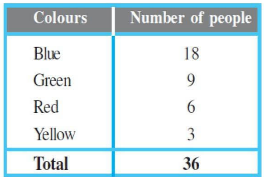# NCERT Solutions for Class 8 Maths Chapter 5 Data Handling

NCERT solutions for class 8 maths chapter 5 Data Handling - In our daily life, we come across various types of data through the newspaper, television, social media which are related to many things like sports, economy, GDP, etc. Also, you must have seen these data are represented in the graphs, charts, and in the tabular form. Why we need data? What is the purpose of representing these data into a chart or in graph form? In CBSE NCERT solutions for class 8 maths chapter 5 data handling, you will get answers to all these questions. Basically, data is the collection of information and observations which can be used to make some important decisions. In this chapter, you will learn to represent data in organized form so that its interpretation and analysis become easier. You will get to know about types of graphs and charts to represent data and some are listed below.

• Tabular Chart- The most simple form of data presentation is Tabular chart where data is always represented in matrix form which is most easy to the readable form of data representation.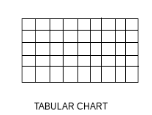• Pie Chart - Pie Chart is the chart where the data represented either in percentage or in the angle made in the middle by that particular sector of the circle.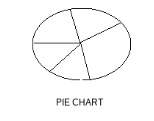• Bar Graph - It is the most preferred method of data representation where data always present in bars and multiple bars makes a bar graph.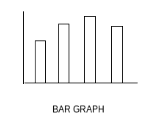• Line Graph - Line Graph is the extended and updated version of Bar Graph where the top of every bar is connected to the next bar’s top and the adjoining of these tops makes a line graph.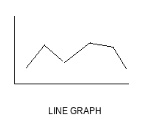There are many questions in the solutions of NCERT for class 8 maths chapter 5 data handling where you will get to know about the use of different types of graphs for different types of data based on the need. There are 3 exercises with 16 questions in this chapter. You will get detailed explanations of these questions in the CBSE NCERT solutions for class 8 maths chapter 5 data handling. Check NCERT solutions from class 6 to 12 to perform well in the exam.

Solutions of NCERT for class 8 maths chapter 5 Data Handling Topic: Looking For Infortmation

Q1 Draw an appropriate graph to represent the given information.

 Month July August September October November December Number of  Watches sold 1000 1500 1500 2000 2500 1500The above given graph represents the data given in the table.

 Month July August September October November December Number of  Watches sold 1000 1500 1500 2000 2500 1500

 Children Who prefer School  A School  B School C Walking 40 55 15 Cycling 45 25 35Above graph represents the data shown in the table.

 Children Who prefer School  A School  B School C Walking 40 55 15 Cycling 45 25 35

Percentage wins in ODI by 8 top cricket teams

 Team From Champions Trophy to World Cup-06 Last 10  ODI in 07 South Africa Australia Sri Lanka New Zealand England Pakistan West Indis IndiaThe above graph represents the data given in table.

 Team From Champions Trophy to World Cup-06 Last 10  ODI in 07 South Africa Australia Sri Lanka New Zealand England Pakistan West Indis India

NCERT solutions for class 8 maths chapter 5 Data Handling Topic: Organising Data

dog, cat, cat, fish, cat, rabbit, dog, cat, rabbit, dog, cat, dog, dog, dog, cat, cow, fish, rabbit, dog, cat, dog, cat, cat, dog, rabbit, cat, fish, dog. Make a frequency distribution table for the same.

The above data can be represented as shown below:

 Animals Tally marks Number of animals Cat 10 Dog 10 Fish 3 Cow 1 Rabbit 4

## Solutions for NCERT class 8 maths chapter 5 Data Handling Topic: Grouping Data

What is the size of the class intervals?

Frequency Distribution of Daily Income of 550 workers of a factory

Table 5.3

 Class Interval    (Daily Income in Rs.) Frequency   (Number of workers) Total

(i) The size of the class intervals =

 Class Interval    (Daily Income in Rs.) Frequency   (Number of workers) Total

Which class has the highest frequency?

Frequency Distribution of Daily Income of 550 workers of a factory

Table 5.3

 Class Interval    (Daily Income in Rs.) Frequency   (Number of workers) Total

(ii) The class which has the highest frequency =  and the highest frequency is

 Class Interval    (Daily Income in Rs.) Frequency   (Number of workers) Total

Which class has the lowest frequency?

Frequency Distribution of Daily Income of 550 workers of a factory

Table 5.3

 Class Interval    (Daily Income in Rs.) Frequency   (Number of workers) Total

(iii) The class which has the lowest frequency =  and lowest frequency is 20.

 Class Interval    (Daily Income in Rs.) Frequency   (Number of workers) Total

Frequency Distribution of Daily Income of 550 workers of a factory

Table 5.3

 Class Interval    (Daily Income in Rs.) Frequency   (Number of workers) Total

(iv) The upper limit of the class interval 250-275 is 275 and frequency is 35.

 Class Interval    (Daily Income in Rs.) Frequency   (Number of workers) Total

Which two classes have the same frequency?

Frequency Distribution of Daily Income of 550 workers of a factory

Table 5.3

 Class Interval    (Daily Income in Rs.) Frequency   (Number of workers) Total

(v) The two classes which have the same frequency are  and   also the frequency is 55.

,   and so on.

The frequency distribution table for the data given is :

 intervels students total =

## Solutions for NCERT class 8 maths chapter 5 Data Handling Excercise: Bars With A Difference

What information is being given by the histogram?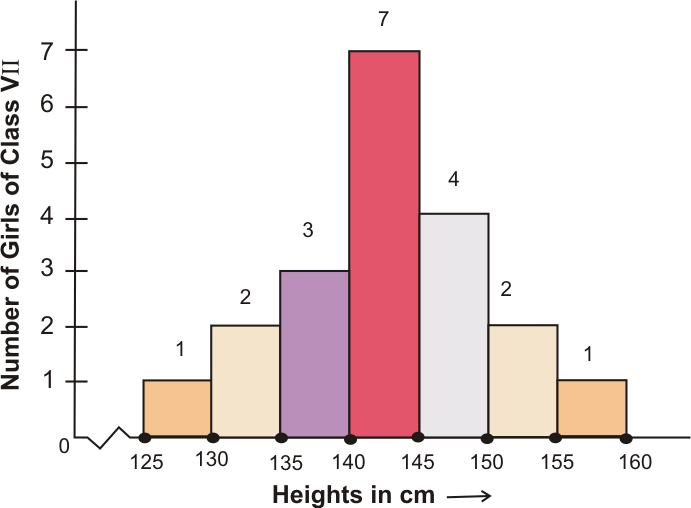The above histogram represents the number of girls as y-axis and heights in cm as x-axis.

Q1 (ii) Observe the histogram (Fig 5.3) and answer the questions given below.

Which group contains maximum girls?(ii) The group which contains maximum girls is(iii) The girls having a height of and more areQ1 (iv) Observe the histogram (Fig 5.3) and answer the questions given below.

If we divide the girls into the following three categories, how many would there be in each?

and more                     —  Group A

to less than    — Group B

Less than                      — Group Cand more                          — Group A =

to less than        — Group B =

Less than                            — Group C =## NCERT solutions for class 8 maths chapter 5 Data Handling Excercise: 5.1

The number of letters for different areas in a postman’s bag.

Give reasons for each.

(a) The number of letters for different areas in a postman’s bag.

Histogram can be represented for the data which can be represented as manner of intervals.The number of letters for different areas in a postman’s bag cannot be represented in form of interval so this cannot be represented using histogram.

The height of competitors in an athletics meet.

(b) The height of competitors in an athletics meet.

Histogram can be represented for the data which can be represented as manner of intervals.The height of competitors in an athletics meet can be represented in form of interval so this can be represented using histogram.

The number of cassettes produced by  companies.

(c) The number of cassettes produced by  companies.

Histogram can be represented for the data which can be represented as manner of intervals.The number of cassettes produced by  companies cannot be represented in form of interval so this cannot be represented using histogram.

The number of passengers boarding trains from to at a station.

(d) The number of passengers boarding trains from to at a station.

Histogram can be represented for the data which can be represented as manner of intervals.The number of passengers boarding trains from to at a station can be represented in form of interval so this can be represented using histogram.

Make a frequency distribution table using tally marks. Draw a bar graph to illustrate it.

The frequency distribution table using tally marks is as shown :

 Shopper Tally marks Number of shoppers W 28 M 15 B 5 G 12 60

The graph is as shown :Using tally marks make a frequency table with intervals as 800–810, 810–820 and so on.

Using tally marks a frequency table with intervals as 800–810, 810–820 and so on is as shown:

 Intervals Tally MARKS Frequency

Which group has the maximum number of workers?

(i) The group which has the maximum number of workers isQ4 (ii) Draw a histogram for the frequency table made for the data in Question 3, and answer the following questions.

How many workers earn   and more?

(ii) The workers earning   and more are 1 + 3 + 1 +1 + 4 = 10How many workers earn less than  ?

(iii) The workers earning less than   are .

3 + 2 + 1 + 9 + 5 = 20For how many hours did the maximum number of students watch TV?(i) From the graph we observed that the maximum number of students (32) watch TV for 4-5 hours.How many students watched TV for less than hours?(ii) 34 students watched TV for less than hours.The number of students who watched TV for less than 4 hours = sum of  the students who watched TV for (3–4hours) + (2–3hours) + (1–2hours.)

So, the total number of students = 4 + 8 + 22 = 34

How many students spent more than hours in watching TV?(iii) 14 students spent more than hours in watching TV.The number of students who watched TV for >5 hours =  number of students who watched TV for (5 – 6 hours) + (6 – 7 hours).

So the no. of students = 8 + 6 = 14

## Solutions of NCERT for class 8 maths chapter 5 Data Handling Topic: Circle Graph or Pie Chartof the fraction of the circle represent girls and other   represent boys of class.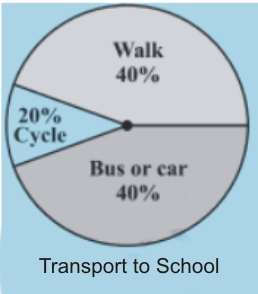The circle represents that  students use cycle for transportation, walk and  use bus or car for transportation.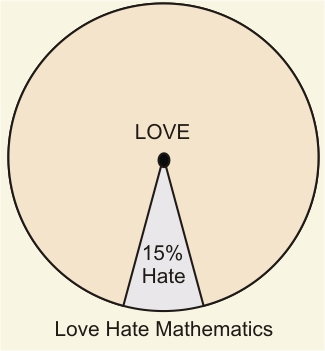(iii)The circle represents that  students love and  students hate Mathematics.

Which type of programmes are viewed the most?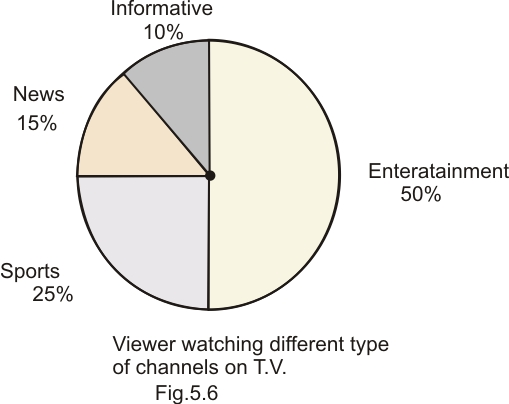(i) Entertainment programmes are viewed the most i.e. .Informative and News together have a number of viewers equal to those watching sports channels.## Solutions for NCERT class 8 maths chapter 5 Data Handling Excercise: Drawing Pie Chart

The time spent by a child during a day.

Sleep —

School —

Homework —

Play —

Others —

Pie chart of the data is given below:## Solutions of NCERT for class 8 maths chapter 5 Data Handling Excercise: Drawing Pie Chart

Production of food grains of a state.

 Year 2001 2002 2003 2004 2005 2006 Production (in lakh tons) 60 50 70 55 80 85

Data can be represented as shown below as bar graph.Choice of food for a group of people.

 Favourite food Number of people North Indian South Indian Chinese Others Total

The given data can be represented as shown below as pie chart.The daily income of a group of factory workers

 Daily Income (in Rupees) Number of workers (In a factory) Total

The data can be represented as shown below as bar graph.## NCERT solutions for class 8 maths chapter 5 Data Handling Excercise: 5.2

(i) If 20 people liked classical music, how many young people were surveyed?

(ii) Which type of music is liked by the maximum number of people?

(iii) If a cassette company were to make 1000 CD’s, how many of each type would they make?(i)If 20 people liked classical music

Total number of people =  =  people.

(ii)  Maximum number of people light music i.e..

(iii) If a cassette company were to make 1000 CD’s, then

Light music CD's =

Folk music CD's =

Classical music CD's =

Semi classical music CD's =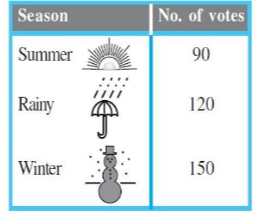(i) Which season got the most votes?

(ii) Find the central angle of each sector.

(iii) Draw a pie chart to show this information.(i) Winter season got the most votes.

(ii)  Total Votes = 90+120+150 = 360

central angle of summer =   =

central angle of rainy =   =

central angle of winter =  =

(iii)The table shows the colours preferred by a group of people.